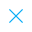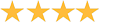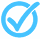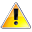Call Support +91-85588-96644

Keep me logged in
You can't leave Captcha Code empty
By submitting this form, you agree to the Terms & Privacy Policy.
ORTests given# Equation of Line By TCY All tests

4.0Questions

Time

Highest
score

Level

## ENGLISH

Language

Topics Covered:Coordinate GeometryEquation of a LineSlope of a LineSlopes of Parallel LinesVarious Forms of the Equation a LineDrawing Inferences from the Equation of a LineEquation of a Line passing through a Given Point Parallel to a Given LineEquation of a Line passing through a Given Point Perpendicular to a Given Line